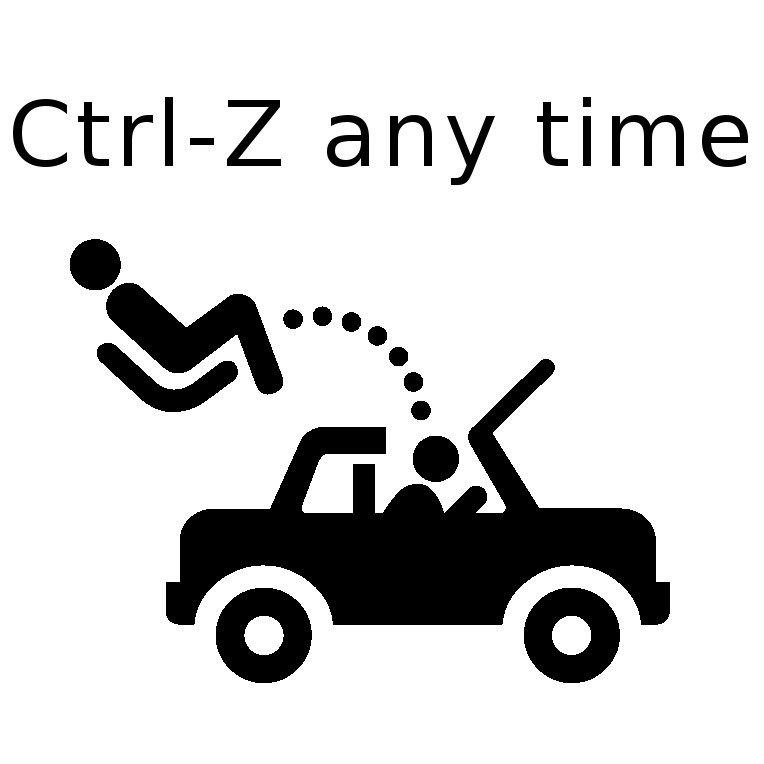# MEI A level maths H640 2018 paper 1: interactive! (with mouse)

### Hover the mouse over a vector for guidance.ARecommended Resource

Main page - lots of interactive A level mechanics

7.(i) Ignore moments, just resolve horizontally: equilibrium means F must equal the sum total of the other two.

(ii) Now hover the mouse near but not quite where the stems of the two rotatable moments join (so that a grey sausage shape is visible, and also a grey text message inviting you to left-click and drag). Thus drag the pair of moments so their join is at the top of the rod at the point of action of the 30N force. See how equilibrium means that the two opposed moments, one clockwise and one anti, must be equal. Solve for x, the distance to the point of action of F.

Your browser does not support the HTML5 canvas tag.

load a saved work file or a background image file:

11.

(i) It's a good idea always to start by collecting all the forces acting at a point and considering their directions. Acting on block A there are only two: tension and weight (no friction).

So, resolving parallel to the slope, the tension must be equal to an appropriate component of the weight. To see how to get the correct trigonometry for the problem, first hover the mouse near the join of the three vectors on the far right - so that a grey sausage shape (not a circle) is visible - then left click and drag the whole shape so that the join is on block A.

Now click on the grey circle at the join and drag to play around. For example drag the join so that the initially vertical red (resultant) arrow is horizontal. Try to see how the "big and little" angles in the 60-30 triangle in block A's part of the diagram show you how to draw a vector parallelogram which is correct just because "big and little" correspond appropriately.

Now press control-Z until the display is back to how it was. See how T must be equal to root-3-over-2 of 4.7g because of the 30-60 trigonometry. (Click near the end of a vector to get dotted lines completing the triangles, which may help to see the trigonometry.)

(ii) First collect the 4 forces acting on B, and prepare to make an equation of all the forces acting parallel to the slope: tension, friction, and an appropriate fraction of the weight.

Again, drag the vector shape (with resultant 4g) so the join of the whole shape is on block B. Click on the grey circle at the join and drag to play around, to sense how you must learn to sketch a similar shape assigning the diagonal (or hypotenuse) to the resultant.

Again, press control-Z to return to normal. See how T must be equal to the sum of the two forces opposing it: 4g sin25, weight component, and then the friction. Then use also that the friction will be μ (the co-efficient) times R the reaction, which in turn must be equal to the other weight component, 4g cos25, as no other forces are acting in that direction.

And again, click near the end of a vector to get dotted lines completing the triangles, to help check the trigonometry. Finally, solve for μ.

Your browser does not support the HTML5 canvas tag.

load a saved work file or a background image file:

14.

(i) Calculate the acceleration as the gradient function of the velocity, as highlighted in the velocity-time graph. Hence present v as a function of t.

(iv) Either choose suitable suvats or use the velocity-time graph to choose suitable area calculations, as shown.

(v) Show that this model gives 11.4 at this time, like the previous one.

(vi) Differentiate 5 + 0.6t2 - 0.05t3 and show that the gradient at t = 8 is zero (like the subsequent acceleration).

(vii) Calculate the areas as indicated.

Your browser does not support the HTML5 canvas tag.

load a saved work file or a background image file:

Main page - more interactive A level mechanics

ARecommended Resource
Garden City Maths - Online tuition!Today! Open a meeting in Zoom and invite by email (below). Or just email me, and I'll help set up.
Balloon Calculus ... Don't integrate - balloontegrate!

email: tom@ballooncalculus.org.